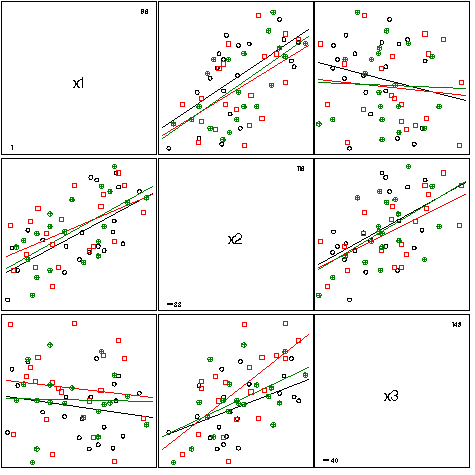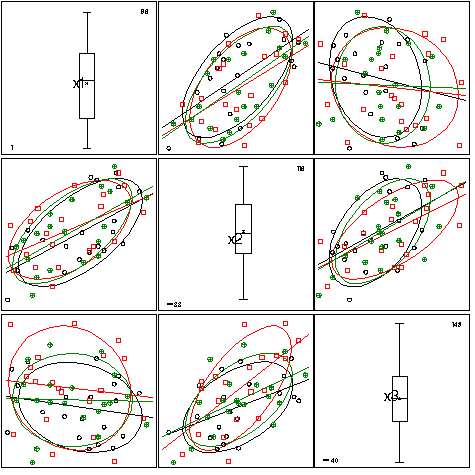scatmat scatmat - scatterplot matrix - all pairwise plots for n variables scatmat

# SAS Macro Programs: scatmat

\$Version: 1.8-0 (24 Jan 2010)
Michael Friendly
York University

## SCATMAT macro (get scatmat.sas)

The SCATMAT macro draws a scatterplot matrix for all pairs of variables specified in the VAR= parameter. The program will not do more than 10 variables. You could easily extend this, but the plots would most likely be too small to see.

If a classification variable is specified with the GROUP= parameter, the value of that variable determines the shape and color of the plotting symbol. The macro GENSYM defines the SYMBOL statements for the different groups, which are assigned according to the sorted value of the grouping variable. The default values for the SYMBOLS= and COLORS= parameters allow for up to eight different plotting symbols and colors. If no GROUP= variable is specified, all observations are plotted using the first symbol and color.

### Dependencies

Depending on options selected, the SCATMAT macro calls several other macros not included here. It is assumed these are stored in an autocall library. If not, you'll have to %include each one you use.
MacroFunctionNeeded
%gdispla device-independent DISPLAY control always
%lowess smoothed lowess curves ANNO = ... LOWESS
%ellipses data ellipses ANNO = ... ELLIPSE
%boxaxis boxplot for diagonal panels ANNO = ... BOX

### Parameters

DATA=_LAST_
Name of the data set to be plotted.
VAR=
List of variables to be plotted. The VAR= variables may be specified as a list of blank-separated names, or as a range of variables in the form X1-X4 or VARA--VARB.
GROUP=
Name of an optional grouping variable used to define the plot symbols and colors.
INTERP=NONE
SYMBOL statement interpolation option. Specifying INTERP=RL gives a fitted linear regression line in each scatterplot.
ANNO=NONE
Provides additional annotations to the diagonal and/or off-diagonal panels of the scatterplot matrix. You can specify one or more of the keywords BOX, ELLIPSE, and LOWESS
• BOX - draws a boxplot showing the distribution of each variable in the diagonal panel for that variable. Requires the boxaxis macro
• ELLIPSE - draws a data ellipse in each off-diagonal panel. Requires the ellipses macro
• LOWESS - draws a smoothed lowess curve in each off-diagonal panel. Requires the lowess macro
SYMBOLS=%str(circle + : \$ = X _ Y)
List of symbols, separated by spaces, to use for plotting points in each of the groups. The i-th element of SYMBOLS is used for group i. If there are more groups than symbols, the available values are reused cyclically.
COLORS=BLACK RED GREEN BLUE BROWN YELLOW ORANGE PURPLE
List of colors to use for each of the groups. If there are g groups, specify g colors. The i-th element of COLORS is used for group i. If there are more groups than colors, the available values are reused cyclically.
NAME=scatmat
Name of the graphic catalog entry
GOUT=GSEG
Name of the graphics catalog used to store the final scatterplot matrix constructed by PROC GREPLAY. The individual plots are stored in WORK.GSEG.

### Example

Generate some random, correlated data, and show the scatterplots with separate regression lines for each group:
```%include macros(scatmat);
data test;
do i=1 to 60;
gp = 1 + mod(i,3);
x1 = round( 100*uniform(12315));
x2 = round( 100*uniform(12315)) + x1 - 50;
x3 = round( 100*uniform(12315)) - x1 + x2;
x4 = round( 100*uniform(12315)) + x1 - x3;
output;
end;

%scatmat(data=test, var=x1-x3, group=gp, interp=rl);
```Show the same data with marginal boxplots, and data ellipses:

```%scatmat(data=test, var=x1-x3, group=gp, interp=rl, anno=BOX ELLIPSE);
```